The ALL() Function in DAX - Excelerator BI

# The ALL() Function in DAX

Level: Intermediate

In this article I am going to write about the ALL() function, show how it works and explain a common use case.  I will also cover the unexpected behaviour that occurs when using the ALL() function in Power BI Desktop – more on that later.

For this article I will use my simplified copy of the Adventure Works database as shown below.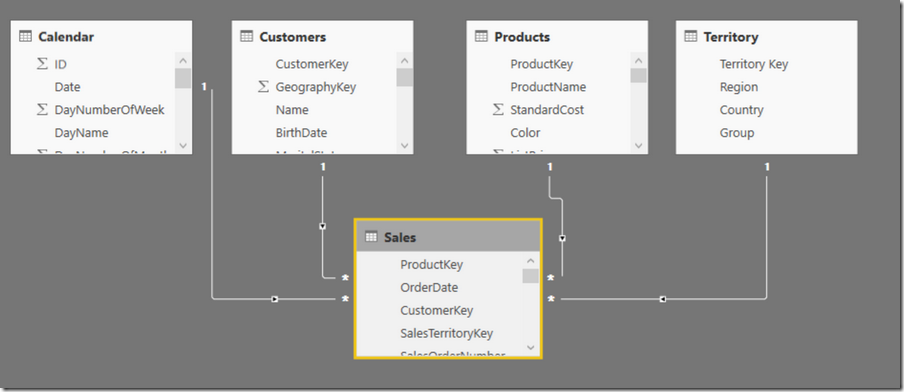## Virtual vs Materialised Tables

The first thing I want to cover is the concept of virtual vs materialised tables.  Normally in DAX when you write a formula that contains a table function (a measure or calculated column) the table is created in memory during execution.  The table is never “materialised” so you can’t see it and it is not stored anywhere.  I refer to these tables as “virtual” tables.  On the other hand it is possible to “materialise” a table in DAX in many ways including

• Loading the table from a data source into Power Pivot/Power BI (materialise to the data model on data load)
• Writing a DAX query and then use the trick I cover here to materialise the table directly into Excel.
• Using the New Table button in Power BI Desktop to materialise the table into the data model after data load as I covered here.
• You can also materialise a table using a Pivot Table in Excel to build a table you want to see.  You should use caution with this approach if you need to materialise many rows of data as a Pivot Table is best used as a summarisation tool, not a large table generation tool.

Virtual tables are a lot harder to get your head around, particularly if you come from the Excel world (SQL pros don’t tend to have this same problem).  For this reason I am going to materialise the tables I produce in this article so you can see them.  But all the way through you need to remember that every table you can “see” below could in theory be used as a virtual table inside another DAX formula.

## ALL() Overview

The ALL() function seems very simple on the surface however it has layers of complexity.  In its most simple usage it is a function that simply returns a table (virtual or materialised).  The syntax for ALL() is as follows

=ALL(TableOrColumn,[Column2],[ColumnN]..)

ALL() will always return a table, not a value.  Because it is a table, you cannot put the result directly into a cell in a Pivot Table or a Matrix.  Think about it, you can’t put a table with (potentially) multiple columns and (potentially) multiple rows into a single cell in a visual – it wont “fit”.

Below are some materialised example tables of simple ALL() usage so you can “see” the results and get an idea of what it is all about. (See what I did there)?  In the examples below I am using DAX as a query language to materialise the tables created by the ALL() function directly into Excel using the materialisation trick I linked to above (again here).### ALL() with a Table Parameter

Test 1 =ALL(Products)

The above formula returns a full copy of the Products table as shown (in part) below.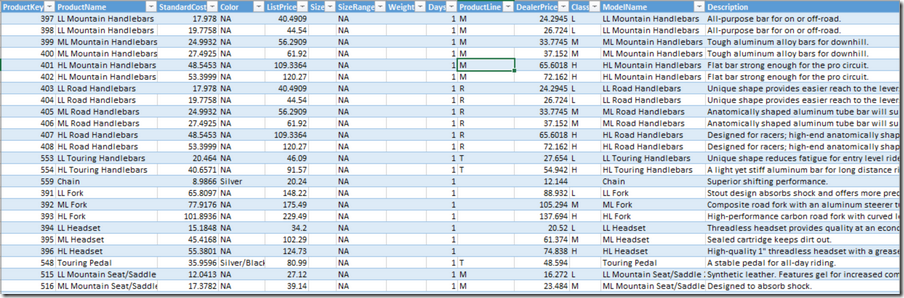Importantly any filter that exists is REMOVED BEFORE producing this table.  So the following DAX query produces exactly the same table.

Test 2=CALCULATETABLE(ALL(Products),Product[Category]=”Clothing”)

If you are not familiar with CALCULATETABLE, it is exactly the same as CALCULATE however it is used when the first parameter returns a table instead of a value.

CALCULATE and CALCULATETABLE always execute the filter portion of the formula first, then the last thing it does is evaluate the expression parameter (the first parameter).  So Test 2 above first applies a filter to the Products table (Products[Category] = “Clothing”) as the filter parameter of CALCUALTETABLE and then the last thing it does is execute the ALL() Parameter.  The ALL() function removes all filters in the current filter context, hence CALCULATETABLE adds a filter and then ALL() removes it.  The same behaviour exists inside a visual (say in a Matrix or Pivot Table) where the visual creates a filter context – ALL() will still remove it. More on that below.

### ALL() with A Column Parameter

There is a second syntax for ALL() where you can use one or more Columns (not a table) as the parameter(s).

Test 3 = ALL(Products[Category]) returns the following table.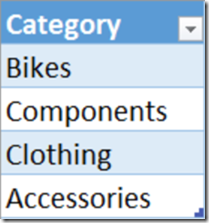It is important to note the following key points.

• This is not a representation of the column from the products table; it is now a Table in its own right.
• In this case I have materialised this table “Table 3” so you can see it, but it doesn’t have to be that way.  The ALL() function can produce this as a virtual table for use inside other DAX formulas. When this happens, the table is never materialised (you can’t see it) but it is real and it is there.
• When this table is created at runtime as a virtual table, it retains lineage to the table from where it originated.  You should think of this as a virtual table that spawns inside the data model and has a relationship to the table from where it came.  See image below.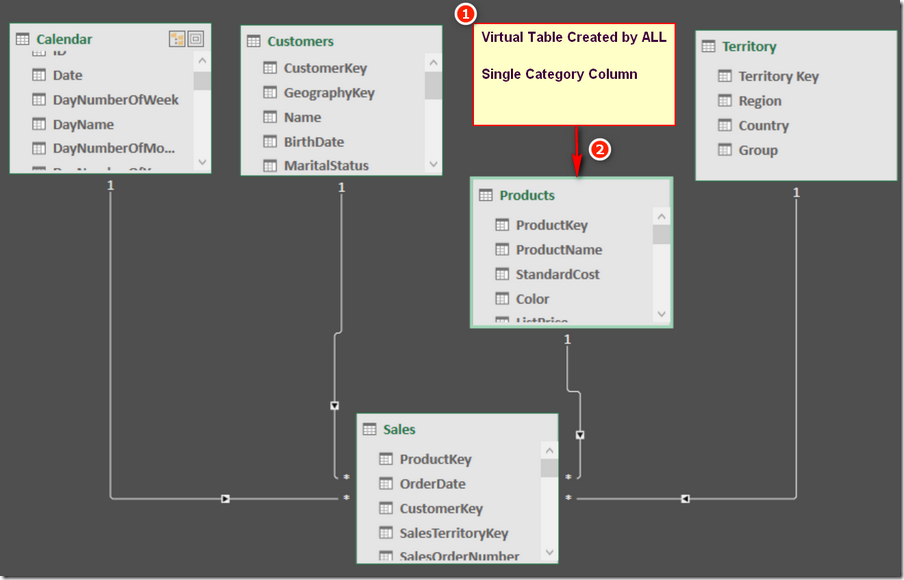The image above is a conceptual illustration of what happens under the hood when you use ALL() inside a formula (ie when the table is not materialised).  When the table is not materialised, the ALL() table (shown as 1) is created in memory at runtime, the table retains a relationship to the table where it was created from (the Products table in this case), and the new table (illustrated conceptually as 1 above) also has a one to many relationship to the table where it came from (Illustrated conceptually as 2 above).  The implication is that you can use this virtual table ALL() anywhere that you can use a materialised table in DAX, and this virtual table will behave exactly as if it were a permanent part of the data model (for the life of the formula).  Once the formula has been evaluated, the virtual table disappears leaving no trace* as if it never existed.

*Actually, it may leave a trace behind. Under certain circumstances Power Pivot will cache the results of a formula and can access that cache for use again for other calculations, but the detail behind this is out of scope for this article.

### ALL() with Multiple Column Parameters

The ALL function can also have multiple column parameters as shown below.

Test 4 = ALL(Products[Category],Products[Color])

The formula Test 4 above produces the following table.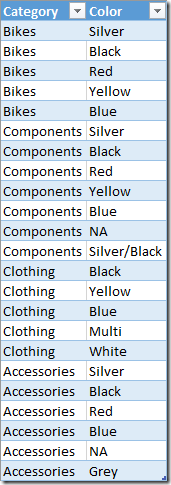The table above contains all of the combinations of Category and Color that exist in the Products table.  There are a couple of points to note

• All the columns inside the ALL() function must come from the same table.
• If you want a table that contains 9 out of a total of 10 columns, you can use the ALLEXCEPT() function instead.
• There is also an ALLSELECTED() function that is slightly different in behaviour (not covered here).## Using ALL() inside a Measure

So far I have always shown materialised copies of an ALL() function so you can “see” what is happening.  However the most common use for the ALL() function (in my experience) is to access the total rows in a visual using a virtual ALL() table.  Take the following Pivot Table as an example.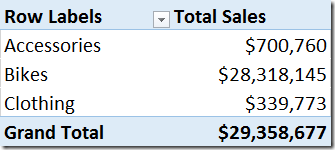Imagine I want to know what % of each of these product categories is of the total sales.  In Excel this would be easy.  You would write a formula that points to the Grand Total, something like this shown below.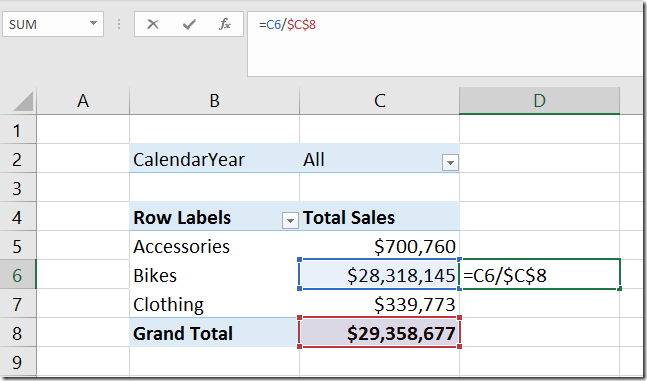Now I am not going to get into the merits of doing it this way (most are bad), just to say that it is “easy” if you want to do it this way, even if it is bad practice (IMO).  In Power Pivot/Power BI, you need a different approach.  The trick to this problem is to create a measure that will materialise the Grand Total value inside the pivot table (or matrix) as shown below.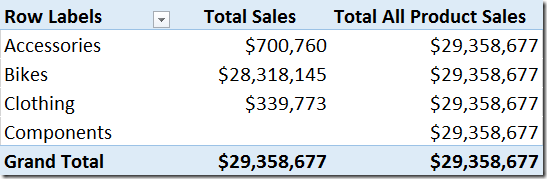This table is pretty easy to produce using the ALL() function as a virtual table inside of CALCULATE as follows.

Total All Product Sales = CALCULATE([Total Sales],ALL(Products))

The way this formula works is as follows:

• CALCULATE always executes the filter parameter(s) first.  In this case the filter parameter is the table function ALL()
• ALL() returns a copy of the Products table with the filters from the current filter context removed.  In this case the 4 rows of the Pivot Table are filtering on Products[Category]. Note that there is no filtering on the Products[Category] column for the Grand Total row, which is why that row always returns the grand total.
• CALCULATE takes the unfiltered copy of the Products table (ie with the filter from the Pivot Table removed), applies this filter to the data model (ie removes the filter) and then evaluates [Total Sales].  This is why the amount \$29m appears on every row in the pivot table for this new measure – because CALCULATE has used ALL() to remove the filters coming from the Pivot Table.

Hopefully now you can see why sometimes we call the ALL() function the “Remove Filters” function (when used with CALCULATE).

Now that you can “see” the Total Sales and Total All Product Sales in the Pivot Table above, hopefully it should now be obvious how to create the % of total sales for each Product Category.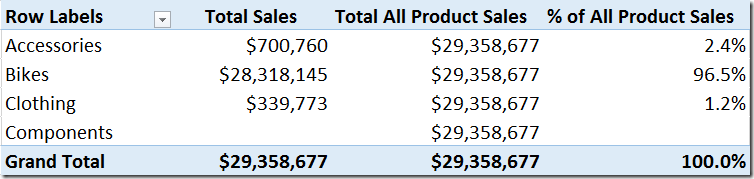% of All Product Sales =DIVIDE([Total Sales],[Total All Product Sales])

## Wait, What about % of Grand Total?

Ok, at this point I hear a few of you say “what about just using the % of Grand Total feature” of Pivot Tables.  Well you are right of course, but there are a few reasons why this may not be the best option.

1. The % of grand total is a visualisation trick – it is not part of the data model.  If you create another pivot table and need the number again, you need to manually apply the visualisation trick again.
2. You can’t use the results of the % of Grand Total visualisation trick inside another measure.  For example, imagine if you want to pay a 10% bonus to all Sales Reps that sell 5% of total sales from Accessories?  You will  need to be able to access the % of Accessories as a number inside another DAX measure if you want to do this, and you can’t do that using the % of Grand Total visualisation trick.

## Beware of Power BI

OK, now for the unexpected behaviour from Power BI.  Take the following Pivot Table and formulas as an example.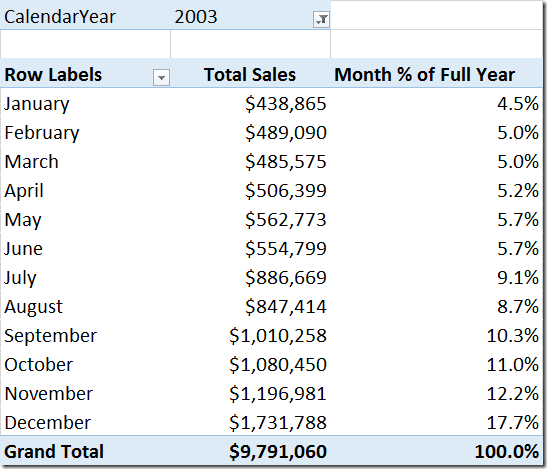```Month % of Full Year =
DIVIDE(
[Total Sales],
CALCULATE([Total Sales], ALL('Calendar'[MonthName]))
)```

A few things to note about the Pivot Table and Measure above.

1. I have deliberately written a more complex measure by adding the CALCUALTE (line 4 above) into a single function.
2. The ALL function in line 4 above removes the filters from the MonthName column only, not the entire table.  For this reason the measure is called “Month % of Full Year” and not “% of Full Year”.
3. The above measure and Pivot Table both work as I expect.

## But What About Power BI Desktop?

Ok, so now when I replicate the exact same measure and visualisation in Power BI Desktop, I get the following (in a Matrix).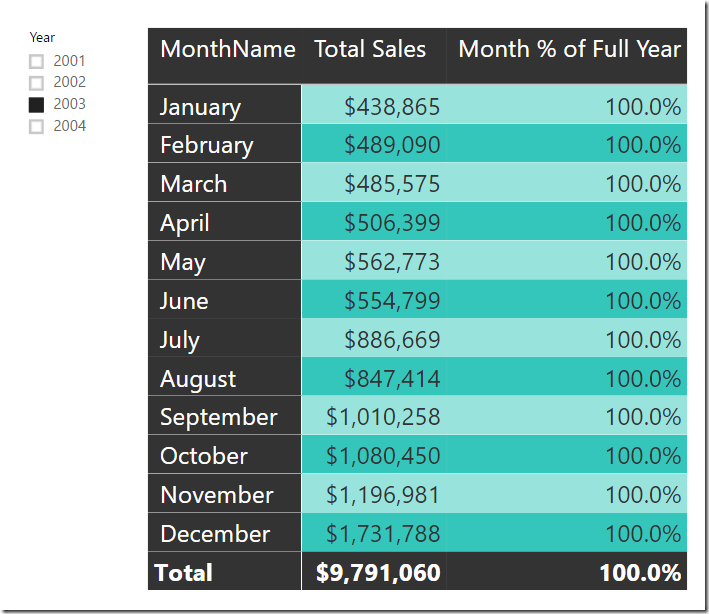Imagine my consternation when I saw this for the first time.  I knew my formula was correct (it is the same one I used in Excel and posted above).  On a hunch I decided to try the following measure instead.

```Month % of Full Year  =
DIVIDE(
[Total Sales],
CALCULATE(
[Total Sales],
ALL('Calendar'[MonthName], 'Calendar'[MonthNumberOfYear])
)
)```

Note the addition of the MonthNumberOfYear column inside my ALL() function above.  This new formula works in Power BI Desktop.

So as you can probably guess by now, I have used the “Sort Columns” feature of Power Pivot and Power BI Desktop to sort the Month Name column in logical month order.  When using Excel, there is no need to remove the filter from this “Sort Column” but it is necessary inside Power BI Desktop.

## Surely This is a Bug I Said

I was pretty sure this was a bug, so I logged it with the Power BI team.  I was shocked by the response I got – “it’s working as designed”.  Well I was not happy with that response, so I sent out a distress call to Marco Russo and asked for his opinion (Marco is the benchmark for what is right and wrong in the world of DAX – in my view).  Marco explained to me that the “Sort by Column” is not actually part of the Power Pivot engine, but is actually part of the visualisation layer.  Said another way, Excel manages “Sort by Column” inside Excel (not Power Pivot) and the visualisation engine does the same thing in Power BI Desktop (not the data modelling engine).  For what ever reason, it is not easy for the Power BI visualisation layer to work the same way as it does in Excel, and there is no plan to change it.   Of course this is not a problem as long as you know, and you know how to handle it – now you do.

### 28 thoughts on “The ALL() Function in DAX”

1.Hi Al. I chatted with some of my brainiac friends, including Phil Seamark and Chris Webb. We agreed we couldn’t work it out, so Phil asked Jeffery Wang (the father of DAX). He says it is a bug! Now we can all relax

1.What is the bug you are referring to?

2.Jason Cockington

This appears to be a response to Al’s question below.
The BUG being referred to is unexpected behaviour with the use of ALL() in a calculated column, where individual ProductKey’s were being returned to each row of the table.
I can confirm that in the current release of Power BI Desktop (April 2023), this BUG no longer exists.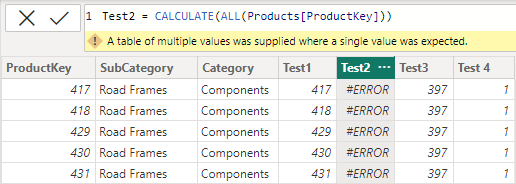2.Hello Matt,
Thank you for the clarification. This article was really helpful. However, I got lost when you were using ALL() inside a measure as shown below:

Total All Product Sales = CALCULATE([Total Sales],ALL(Products))

Now, my question has to do with the [Total Sales]. Is it a column name, or a measure, or something else. If it’s a measure as suspected, then how is the measure calculated.

1.Technically [Total Sales] cannot be a column in this instance because a column can have multiple values and hence cannot be used as the first parameter in CALCULATE. Best practice says never put the table name before the measure name and always put the table name before the column name. I follow best practice, so you can always tell that if there is no table name, it’s a measure.

The measure is calculated based on the underlying measure formula after any filters on the Products table have been removed (the ALL part).
in this case, total sales = SUM(Sales[ExtendedAmount])

3.Thank you for this great explanation.

4.Hi Al. I chatted with some of my brainiac friends, including Phil Seamark and Chris Webb. We agreed we couldn’t work it out, so Phil asked Jeffery Wang (the father of DAX). He says it is a bug! Now we can all relax 🙂

5.Well, technically it is not a bug, but glad it helped anyway.

6.Thank you very much! I did’t know about bug with SortByColumn feature before had read this post. You saved lot of my time

7.This is a super complex topic and it requires a detailed undestanding of how the engine works under the hood. That would be a question for Jes (Oxenskiold) or Marco Russo, maybe also Ozeroth.

8.Hi Matt,
I have the following calculated columns in a ‘Product’ table (ProductKey has no duplicates):
A) ‘Product’[Test 1]=CALCULATE(VALUES(’Product’[ProductKey])) yields the ProductKey per row, as expected
B)‘Product’[Test 2]=CALCULATE(ALL(’Product’[ProductKey])) ALSO yields the ProductKey per row, NOT AS EXPECTED.
C)‘Product’[Test 3]=CALCULATE(COUNTROWS(ALL(’Product’[ProductKey]))) However yields 2517 in all rows (size of the Product table) as expected.
D)‘Product’[Test 4]=CALCULATE(COUNTROWS(VALUES((’Product’[ProductKey])))) yields a 1 in all rows as expected.

My question is, what is going on in ‘Product’[Test 2]? Since we are using ALL(), I would expect the operation to yield an error for returning a table rather than a scalar. If the filter resulting from the context transition is overridden by the ALL(), we would have multiple rows as a result of the CALCULATE. Why is the behavior here different from what we see in C) and D) where the use of ALL does result in different outcomes?

Thank you very much

9.Matt: in regards to your example where you have this:
Test 2=CALCULATETABLE(ALL(Products),Product[Category]=”Clothing”)

So what do you do if you need the ALL to be applied after the filter condition is evaluated? I would have though that you could just use this:
Test 2=CALCULATETABLE(ALLEXCEPT(Products, Products[Category]),Product[Category]=”Clothing”)
…but in a PBI file where I tried this, CALCULATETABLE(ALLEXCEPT ditches the column completely, instead of just ignoring filters on it, as per the question at the following link:

10.RAJKAMAL CHOUDHARY

One of the best description of All, Thanks alot for sharing this.

11.Hi Matt,
In the article, you said that” When the table is not materialised, the ALL() table (shown as 1) is created in memory at runtime, the table retains a relationship to the table where it was created from (the Products table in this case), and the new table (illustrated conceptually as 1 above) also has a one to many relationship to the table where it came from (Illustrated conceptually as 2 above)”.
Could you explain why it’s one to many relationship?
If i’m not wrong, All() returns the entire column in the form of a table hence it is identical with the column (from the original table) so it’s 1 to 1 relationship.

1.No. ALL does not return the entire column. It returns a distinct list of all of the values in the column with filters removed. You can test this using the New Table Button in Power BI desktop. Testing like this can really help your understanding. So given ALL returns a distinct list of the values in the column, the rest should make sense.

1.Thank you for your reply. i tested it as you suggested. you are right 🙂

12.Matt – Thank God you are so Active in the DAX Community

All I can say is thank you for the Power BI Solution. I have faffed about for 4 hours trying to sort this exact issue. I had a gradeset that had a sort by attached and it was being ignored as in the above example. Now it works.

Thanks

Chris

13.Matt, Interesting! I did not know about the need to also clear the filter on the sort by column in Power BI Desktop. I also liked the way you presented the concept of lineage.

One of the ways I use to remind myself of the different behavior of ALL when wrapped around only a table name and when ALL is wrapped around columns from the same table: when ALL is wrapped around only a table name, the DAX engine will return duplicate rows when they exist; however when ALL is wrapped around columns from the same table, the formula engine only returns unique rows.

14.In terms of optimisation of models is it bad to be use ALL (LargeTable) inside a measure? I had thought that ALL just takes filters away but your explanation shows that you are actually bringing a new table into your measure. Does this have a penitently in terms of optimisation of your measures?

1.I have a clear memory of Alberto Ferrari saying “Never filter a table if you can filter a column instead”. So in principle I think yes. However in the case of ALL(Table), it is removing filters rather than adding filters, so I don’t think it would be a train smash. JMO, you would need to test it, but I think it is OK. On the other hand if it was something like
A: SUMX(ALL(Table), expression) or
B: SUMX(ALL(Table[Column]), expression)
and A and B gave the same result, then definitely use B.

15.Very nice article Matt!

I have found that to understand the ALL function(s) it helps to write up the complement-expressions. I.e. ALL(table1(column1) shields the target table from the filter context. The complement expression would be one that is open to the filter context and returns the values of the same column(s).

Shielded from the filter context:
(1) ALL(table1[column1])
(2) ALL(table1[column1], table1[column2] … )
(3) ALL(table1)

Open to the filter context:
(1) VALUES(table1[column1])
(2) SUMMARIZE(VALUES(table1), table1[column1], table1[column2] …)
(3) VALUES(table1)

It also helps in my opinion to write up the equivalent-expressions. I.e. how would you write ALL(table1[column1]) if you had to write an equivalent DAX expression using an appropriate DAX table returning function:

(1) CALCULATETABLE(VALUES(table1[column1]), ALL(table1))
(2) CALCULATETABLE(SUMMARIZE(VALUES(table1), table1[column1], table1[column2] …), ALL(table1))
(3) CALCULATETABLE(VALUES(table1), ALL(table1))

As you can see there is a certain pattern to it.

ALL has a direct sibling called ALLNOBLANKROW that in the same way can be written up as:

Shielded from the filter context:
(1) ALLNOBLANKROW(table1[column1])
(2) doesn’t exist
(3) ALLNOBLANKROW (table1)

Open to the filter context:
(1) DISTINCT(table1[column1])
(2) doesn’t exist
(3) table1

Equivalent-expressions:
(1) CALCULATETABLE(DISTINCT(table1[column1]), ALL (table1))
(2) doesn’t exist
(3) CALCULATETABLE(table1, ALL (table1))
Pleas note that nowhere is DISTINCT(table1) used. Never ever ever use DISTINCT(table1) on a big fact table. DISTINCT(table1) serves a different purpose.

This also goes to illustrate the only 3 ways you can scan a table in DAX. The VALUES and DISTINCT decorators and the naked table reference ‘table1’.

1.The real McCoy:
>This also goes to illustrate the only 3 ways you can scan a table in DAX. The VALUES and DISTINCT decorators and the naked table reference ‘table1’.<

😉

16.17.Nice post, Matt!
A’s far as I can remember, there is also a Date hierarchy pitfall in Power BI too.

18.Steven Doherty

“if only I knew then what I know now”… I always suspected there was something wrong with Power BI. I could never get the All() function to work correctly in certain situations. I’ve also found, through my trial and error process to get things to work that certain visualisations ie. Hierarchy slicer also complicate the way the All() function works as well.

19.Another great post Matt! I appreciate your ability to write about a topic and be able to reach people of all different skill levels. I’m quite familiar with ALL(), but I definitely learned something new with the Power BI “quirk” that you highlighted.

Marco called out another instance of the Sort By “bug”, but I thought it only applied to RANKX()…or due to the row context introduced. Thank you in advance for saving me an hour of troubleshooting!

20.Thanks Matt for explaining ALL in such a simple manner! I always use calculatetable to see a materialise table before I use it in a measure. A very nice article to understand virtual table created in most of the DAX formulas.

Scroll to Top